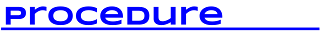## Monday, 16 January 2017

### Equation StripsIn Ontario our grade 7 students are introduced to solving simple equations in the form ax + b = c where the values of a, b and c are whole numbers. We think it's a good idea for them to start by having some sort of visual representation of each equation. In this activity, students are given 16 cards that correspond to 16 equations represented as strips (the top and bottom of the strips represent the left and right sides of the equations). Students solve for x given the strips and then rewrite the algebraic form equation. We originally got this idea from the 2011 Solving Equations Gap Closing resource (pg14).
[Updated Mar 6, 2018 - now both the printable cards and the dynamic websketch have equations in the form 2x - 5 = 19. This puts it beyond the grade 7 expectation but could be an extension or just used for grade 8]
[Updated Dec 19, 2019 - now including a single dynamic Desmos version where you can switch all the versions using sliders as well as a Desmos Activity where students first do some static practice problems and then it finishes with a Challenge Creator where students make their own question for the others to solve]
[Updated May 9th, 2022 - I added a card sort to the Desmos Activity and included a pdf version]• Each group gets a set of 16 cards (24 if you use the cards with minuses)
• Make several copies of the cards on card stock and laminate them so they last longer. You may wish to copy each set onto a different colour so that if they get mixed up you know each set by their colour.
• Cut out the cards so that each group gets a set of 16.1. Each group of 2-3 students gets one full set of 16 cards.
2. Students are to determine the value of x for each card.
3. Once determining x then they should then determine the algebraic expression for each card
4. You can circulate with the solution card to check answers.
5. Once finished you can create your own cards using this web sketch or this Desmos sketch. This allows you to change the coefficients of a, b & c and it generates all four possible configurations. This web sketch assumes that a, b & c will be whole numbers and will not allow any solutions that have x as negative. Once you put your coefficients in then take a screenshot, use the screen capture software of your choice to copy and paste the version you want to use (For Windows use the Snipping Tool, for Chromebooks use Shift CTRL F5, for Macs use Command Shift 4, or iPad use the Home and Sleep buttons together. You can then paste into the word processor of your choice.
6. If using the Desmos Activity, pair students so that they can have conversations about the strips. Be sure to explain how when they create their challenge, they will first have to solve it before they can submit it for the rest of the class to do. When using the Desmos activity, note that the "x's" are missing to make the connections to explicit equations less visible. This is so that students are free from the stigma of actual equations while still solving them. You might want to start with this.
Note that if you want to modify the Desmos activity, you might want to watch this video on how to do it:

Did you use this activity? Do you have a way to make it better? If so tell us in the comment section. Thanks